## Inches to Square Feet Calculator

Use this Inches to Square Feet Calculator to find the square footage of a room:#### 0### How To Calculate Square Feet from Inches?

#### Use the Inches to Square Feet Calculator​ to Calculate The Area Of One Rectangular Room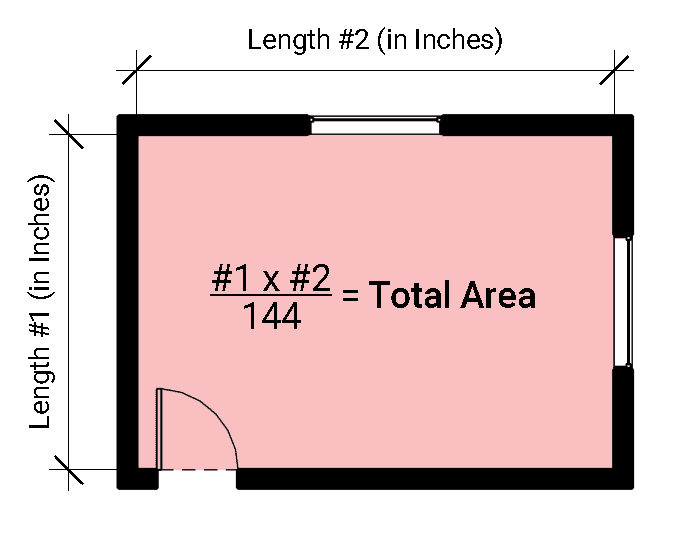To get square feet from inches take the length of one side of a room and multiply it by the length of the other side of the room and divide by 144.  As an equation, it looks like this:

inches of length x inches of length /144 = Area in Square Feet

It doesn’t matter which dimension you use first.

This works well for a rectangular shaped room.  But what if your room is more than just one rectangle?  Continue reading to find out how to calculate the area of other room types.

#### How To Calculate The Area Of A Room With Multiple Spaces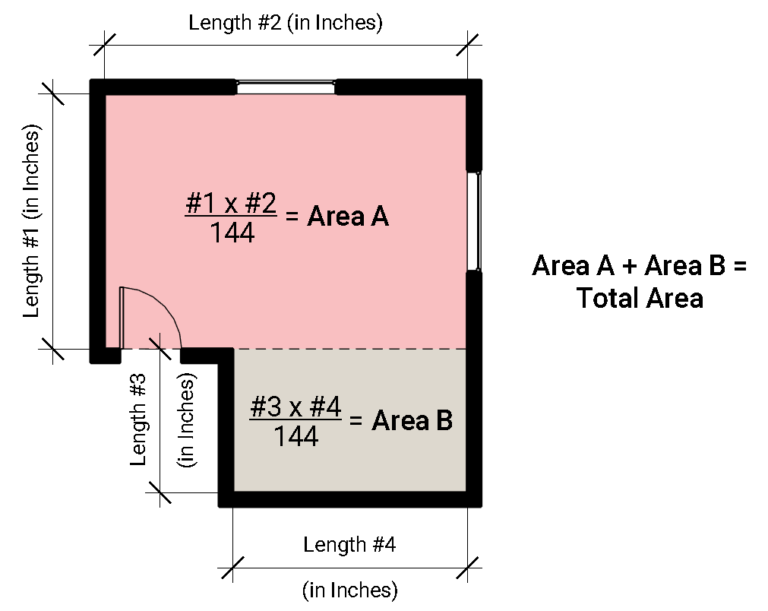If your room is made up of various spaces, break your room up into different rectangles.  Calculate the square feet for each rectangle separately then add up the square feet for each rectangle to find the total area of your room.

The equation looks like this:

Area A + Area B + (as many areas as you have) = Total Area

#### Calculate The Area of A Triangular Room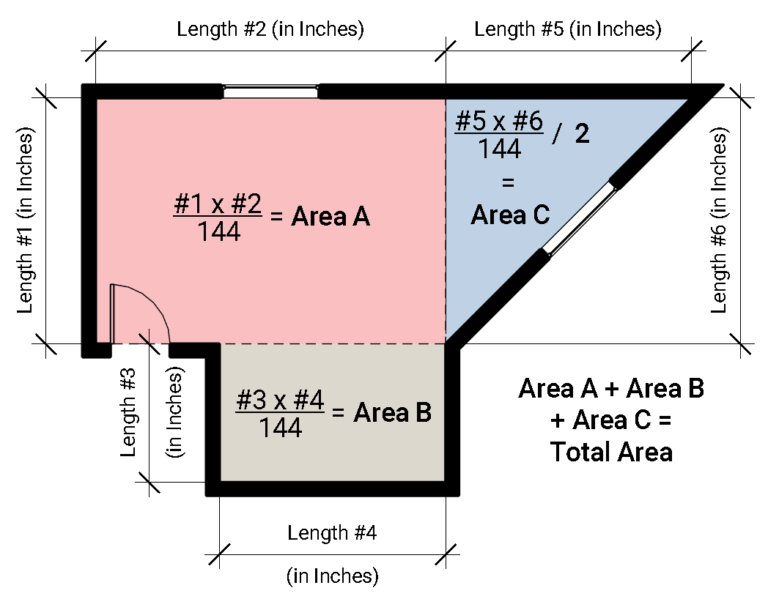What if your room contains triangle shaped spaces?  If your room has a triangle shaped space, measure its two straight lengths and then calculate the area.  Then divide that area by 2.  Your result is the area of the triangle shaped space.

The equation looks like this:

inches of length x inches of length /144/2 = Area of Triangle

Then add that area to all of the rectangle shaped spaces in the room to find your total area.

### Why Calculate Square Footage from Inches?

###### Why not? Calculating square feet from inches can be a good way to go: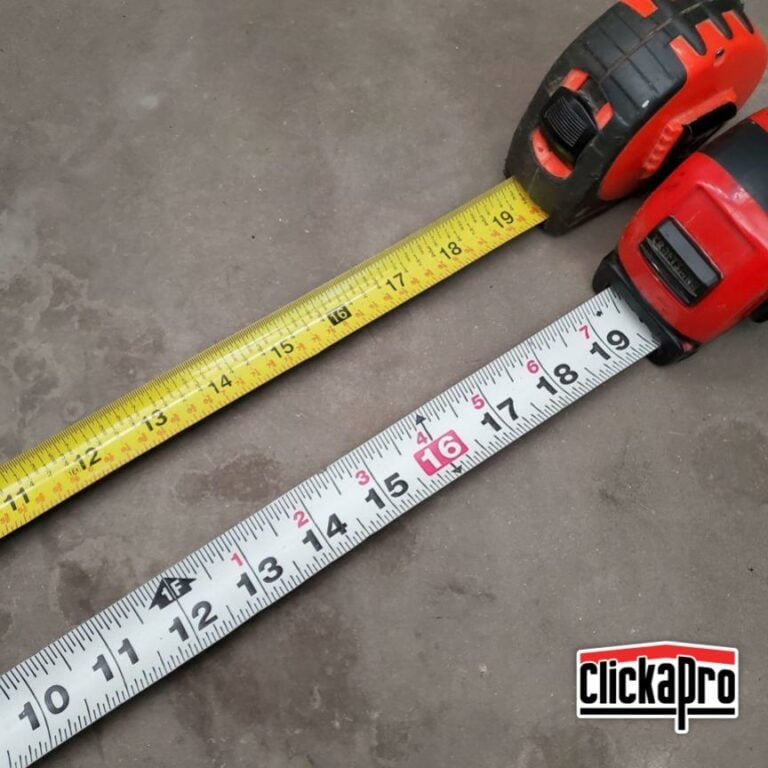There are 4 main reasons to calculate the area of a room from lengths measured in inches:

1.  Your tape measure may only have inches.  Most tape measures have inches starting from 1 inch and counting up.  Many tape measures also have feet marks and count up in inches from 1-11 starting from each foot.  But some tape measures ONLY have numbers for inches.  If that’s the kind of tape measure you have, well, you’re probably going to measure in inches.

2.  But that’s not a problem because there are other reasons to calculate area from inches.  One of the best reasons is that it is easier to calculate the area.  Unless you have an Architect’s calculator that accepts feet and inches, your first step to find the area is to convert your measurement into inches.  So why not just start with inches?

3.  You may be new to feet and inches.  So measuring in inches might be the easiest way to get your project done.

4.  It can be mentally easier to remember that your room is 97″ long than to remember that it is 8′-1″ long.

### How Do I Use The Tick Marks on a Tape Measure?

###### Tick marks can be converted to decimals or fractions:

Each inch on an imperial tape measure is broken up into tick marks.  The largest tick mark in the center represents half an inch.  It’s decimal number is 0.5.

The next largest tick marks are for quarter inches. The first of these tick marks represents 1/4″ and the second is for 3/4″

The next size down tick marks represent 1/8″ increments.

And the smallest tick marks are for 1/16″ increments.

Each tick mark can be converted to a decimal by dividing its fraction.  For example:

The smallest tick mark, 1/16″ would be:

1 divided by 16 = 0.0625″

The largest tick mark in the middle, 1/2″ would be:

1 divided by 2 = 0.5″

This diagram shows each tick mark as both a  decimal and as a fraction.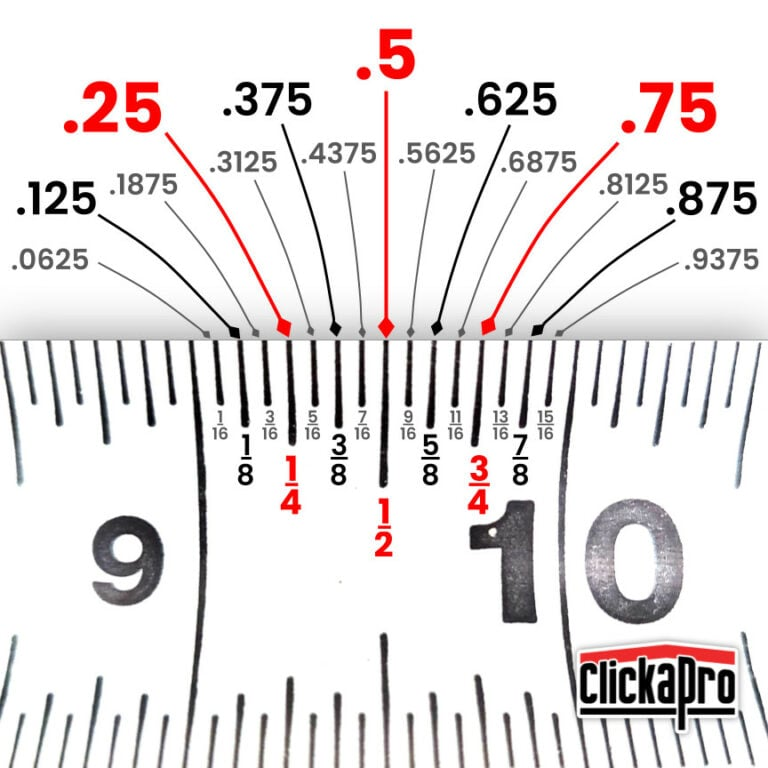Shopping Cart
Scroll to Top